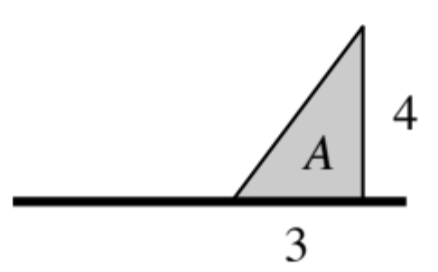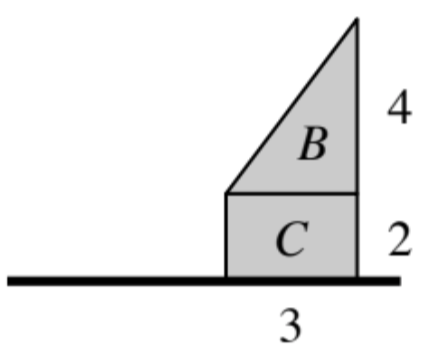### Home > APCALC > Chapter 4 > Lesson 4.1.1 > Problem4-12

4-12.

Khi thinks that when the flags at right are rotated, the $\left(\text{volume of }A\right)+$$\left(\text{volume of }C\right)=$$\left(\text{volume of}\left(B+C\right)\right)$.

Decide if Khi is correct. If he is incorrect, explain the error in his logic.

Start by visualizing the terms in Khi's equation: What does Volume $A$ and Volume $C$ look like? What does Volume $\left(B + C\right)$ look like?

Volume $A$ is cone with radius $4$. Volume $C$ is a cylinder with radius $2$. Each is a separate 3D solid whose volumes are added together after they are computed: $V = [\text{cone with } r = 4] + [\text{cylinder with } r = 2]$

Try computing the volume of each:

$\text{Volume } A + \text{Volume } C = \text{cone} + \text{cylinder}$

$=\frac{1}{3}\pi r^{2}h \ + \ \pi r^{2}h$

$\text{Volume} \left(B + C\right) = \text{Big Cone} − \text{Small Cone}$

$=\frac{1}{3}\pi r^{2}h \ - \ \frac{1}{3}\pi r^{2}h$

Evaluate and compare.

Volume $\left(B + C\right)$ is a single 3D solid that looks like a cone with its top cut off. To compute its volume, it must be dissected into pieces:
$V = [\text{large cone with } r = 6] − [\text{small cone with } r = 2]$

Use the eTool below to visualize this problem.
Click on the link to the right to view the full version of the eTool: Calc 4-12 eTool